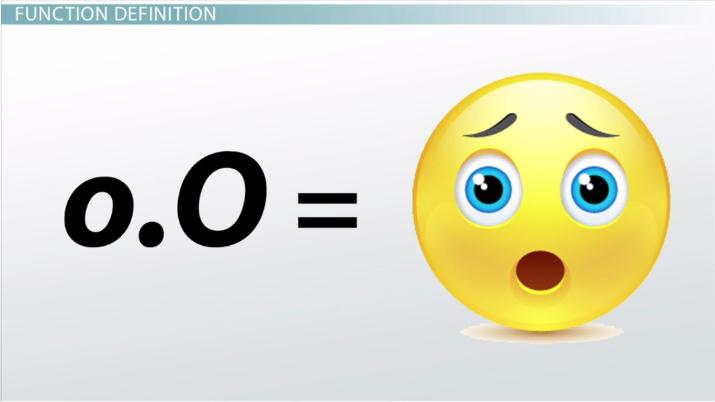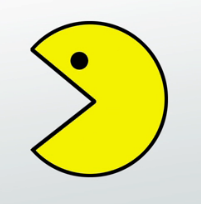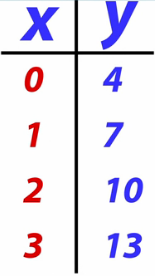Composing Functions: Definition and Examples

Lesson Transcript
Instructor: John Sepanski

John has taught 6th Grade Mathematics through Geometry and has a Master's degree in Education

A function represents the relationship between different variables and it is used to represent some examples of real-world phenomena. Learn the definition of a function, how to compose functions, the domain, range, and notation of a function, and how to use a function table. Updated: 10/09/2021

Composing Functions

Have you ever used emoticons?

You know, emoticons are facial expressions that you can use when you text or type on some apps.

For instance, if you type, :), you will getOr if you type ;), you will getSome people get so used to using emoticons that it's just something that they naturally type.

As a matter of fact, it could be said thatIs the outcome of typing :).

An input of ;), will produce an output ofMathematical functions are exactly like that.An error occurred trying to load this video.

Try refreshing the page, or contact customer support.

Coming up next: Model a Linear Relationship Between Two Quantities

You're on a roll. Keep up the good work!

Replay
Your next lesson will play in 10 seconds
• 0:06 Composing Functions
• 0:50 Function Definition
• 2:04 Domain, Range and…
• 3:15 Function Table
• 5:12 Lesson Summary
Save Save

Want to watch this again later?

Timeline
Autoplay
Autoplay
Speed Speed

Function Definition

A function is a mathematical relationship where there is exactly one output for each input.

The set of the input values is called the domain and the set of output values is called the range.

Maybe this is going a little too fast, and you're beginning to feel a little o.ODon't worry because I'm going to make it so easy that you're going to <3 math!

Let's examine the function definition more closely.

A function is a mathematical relationship where there is exactly one output for each input. Think about the word, 'relationship.' Maybe some of you are in a relationship. A relationship is literally a state or status of being connected. In mathematics, this state of being connected is often expressed through the use of an equals sign.

So that must mean that an equals sign is just a mathematician's way of typing <3.

Back to the definition.

A function is a mathematical relationship where there is exactly one output for each input.

Input. That's like the text you type into your device to produce an emoticon. The emoticon is the output.

Domain, Range and Function Notation

In math, the input and the output are identified with special names: domain and range. Remember, the domain is the set of all input values, and the range is the set of all output values.

Let's see how these fit into a mathematical expression.

:vTo display functions, we use a system of mathematical symbols known as functional notation. When we write in functional notation, it's kind of like writing in text. We use specific expressions to mean certain things. For example, we write functions like this:

f(x) = 2x + 2

This is read, f of x equals 2x + 2.

So, f of x is the range.

x itself is the domain.

In other words, if we insert the domain into a function, as a result of the function, we get the range.

Sometimes the range is indicated with the variable y.

So, sometimes a function like f(x) = 3x + 4 is written as y = 3x + 4.

Function Tables

A math function table can be used to plot the possible outcomes of a function. Function tables are sometimes called T-Tables.This particular table is for the function:

y = 3x + 4

If you input 0 into the function in the place of x, the output (or y value) is 4. If you input 1 into the function, the output is 7. If you input 2 into the function, the output is 10. And if you input 3 into the function, the output is 13.

To unlock this lesson you must be a Study.com Member.

Register to view this lesson

Are you a student or a teacher?